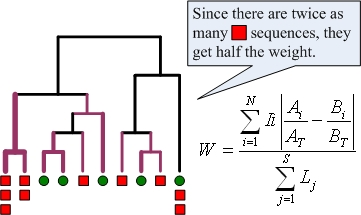# Weighted UniFrac algorithm

The weighted unifrac algorithm is implemented within mothur as the unifrac.weighted command. The algorithm represents an attempt to make a quantitative beta diversity measurement that can be seen as an extension of unweighted UniFrac . Lozupone et al. (2006) have shown that it is beneficial to apply both quantitative (weighted UniFrac) and qualitative (unweighted UniFrac) measures to a data set as they can lead to different conclusions about the similarity of microbial communities and what factors are driving microbial biodiversity. As shown by Schloss (2008) , this is not a reliable approach to measuring similarity; it is best used for hypothesis testing.

The image below illustrates the calculation of weighted UniFrac when applied to two microbial communities identified by red squares and green circles. Branches are weighted to account for the relative proportion of sequences from each community that descend from the branch. The branches colored purple are those that lead to non-zero values in the numerator. To account for varying rates of evolution between sequences, the weighted UniFrac measure is normalized such that each sequence contributes equally to the distance calculated.(See discussion page for possible correction.)

Formally, the measure is calculated using the formula given above where N is the number of nodes in the tree, S is the number of sequences represented by the tree, li is the branch length between node i and its ‘parent,’ Lj is the total branch length from the root to the tip of the tree for sequence j, Ai and Bi are the number of sequences from communities A and B that descend from the node, and AT and BT are the total number of sequences from communities A and B.

Weighted UniFrac can be applied to multiple communities by first constructing a single tree consisting of all sequences. The distance between any pair of communities is calculated by pruning the tree so it contains only sequences from these communities. Like unweighted Unifrac, a random (Monte Carlo) permutation test can be performed to test whether or not the distance between two communities is greater than expected by chance alone.